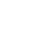# Remove empty elements from an Array using PHP

Mar 14, 2014

Last updated on May 23rd, 2016 at 04:44 pm

## Remove empty elements from an Array using PHP

In the variable data array I have given 8 data elements.
Let \$a, \$b, \$c, \$d,\$e contains data and others that is \$f, \$g, \$h are empty so we will get the new array size as 5 by using the sizeof() function in PHP.

```<?php
\$data=array("\$a", "\$b", "\$c", "\$d", "\$e" , "\$f" ,"\$g" , "\$h");
//print_r(\$data);
function array_trim(\$data)
{
\$j = 0;
for (\$i = 0; \$i<count(\$data); \$i++)
{ if (\$data[\$i] != "")
{ \$b[\$j++] = \$data[\$i];
}
}
return \$b;
}
\$new_size=array_trim(\$data);
```

#### Related Post

##### One thought on “Remove empty elements from an Array using PHP”
1.samarubanok says:

good..